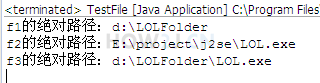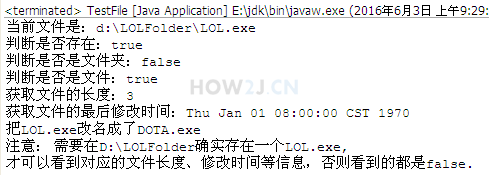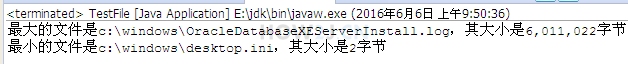14分55秒

## 创建一个文件对象package file; import java.io.File; public class TestFile { public static void main(String[] args) { // 绝对路径 File f1 = new File("d:/LOLFolder"); System.out.println("f1的绝对路径：" + f1.getAbsolutePath()); // 相对路径,相对于工作目录，如果在eclipse中，就是项目目录 File f2 = new File("LOL.exe"); System.out.println("f2的绝对路径：" + f2.getAbsolutePath()); // 把f1作为父目录创建文件对象 File f3 = new File(f1, "LOL.exe"); System.out.println("f3的绝对路径：" + f3.getAbsolutePath()); } }
```package file;

import java.io.File;

public class TestFile {

public static void main(String[] args) {
// 绝对路径
File f1 = new File("d:/LOLFolder");
System.out.println("f1的绝对路径：" + f1.getAbsolutePath());
// 相对路径,相对于工作目录，如果在eclipse中，就是项目目录
File f2 = new File("LOL.exe");
System.out.println("f2的绝对路径：" + f2.getAbsolutePath());

// 把f1作为父目录创建文件对象
File f3 = new File(f1, "LOL.exe");

System.out.println("f3的绝对路径：" + f3.getAbsolutePath());
}
}
```

## 文件常用方法1package file; import java.io.File; import java.util.Date; public class TestFile { public static void main(String[] args) { File f = new File("d:/LOLFolder/LOL.exe"); System.out.println("当前文件是：" +f); //文件是否存在 System.out.println("判断是否存在："+f.exists()); //是否是文件夹 System.out.println("判断是否是文件夹："+f.isDirectory()); //是否是文件（非文件夹） System.out.println("判断是否是文件："+f.isFile()); //文件长度 System.out.println("获取文件的长度："+f.length()); //文件最后修改时间 long time = f.lastModified(); Date d = new Date(time); System.out.println("获取文件的最后修改时间："+d); //设置文件修改时间为1970.1.1 08:00:00 f.setLastModified(0); //文件重命名 File f2 =new File("d:/LOLFolder/DOTA.exe"); f.renameTo(f2); System.out.println("把LOL.exe改名成了DOTA.exe"); System.out.println("注意： 需要在D:\\LOLFolder确实存在一个LOL.exe,\r\n才可以看到对应的文件长度、修改时间等信息"); } }

## 文件常用方法2

package file; import java.io.File; import java.io.IOException; public class TestFile { public static void main(String[] args) throws IOException { File f = new File("d:/LOLFolder/skin/garen.ski"); // 以字符串数组的形式，返回当前文件夹下的所有文件（不包含子文件及子文件夹） f.list(); // 以文件数组的形式，返回当前文件夹下的所有文件（不包含子文件及子文件夹） File[]fs= f.listFiles(); // 以字符串形式返回获取所在文件夹 f.getParent(); // 以文件形式返回获取所在文件夹 f.getParentFile(); // 创建文件夹，如果父文件夹skin不存在，创建就无效 f.mkdir(); // 创建文件夹，如果父文件夹skin不存在，就会创建父文件夹 f.mkdirs(); // 创建一个空文件,如果父文件夹skin不存在，就会抛出异常 f.createNewFile(); // 所以创建一个空文件之前，通常都会创建父目录 f.getParentFile().mkdirs(); // 列出所有的盘符c: d: e: 等等 f.listRoots(); // 刪除文件 f.delete(); // JVM结束的时候，刪除文件，常用于临时文件的删除 f.deleteOnExit(); } }

## 练习-遍历文件夹

姿势不对,事倍功半! 点击查看做练习的正确姿势## 答案-遍历文件夹3分6秒 本视频采用html5方式播放，如无法正常播放，请将浏览器升级至最新版本，推荐火狐，chrome，360浏览器。 如果装有迅雷，播放视频呈现直接下载状态，请调整 迅雷系统设置-基本设置-启动-监视全部浏览器 (去掉这个选项)。 chrome 的 视频下载插件会影响播放，如 IDM 等，请关闭或者切换其他浏览器

package file; import java.io.File; public class TestFile { public static void main(String[] args) { File f = new File("c:\\windows"); File[] fs = f.listFiles(); if(null==fs) return; long minSize = Integer.MAX_VALUE; long maxSize = 0; File minFile = null; File maxFile = null; for (File file : fs) { if(file.isDirectory()) continue; if(file.length()>maxSize){ maxSize = file.length(); maxFile = file; } if(file.length()!=0 && file.length()<minSize){ minSize = file.length(); minFile = file; } } System.out.printf("最大的文件是%s，其大小是%,d字节%n",maxFile.getAbsoluteFile(),maxFile.length()); System.out.printf("最小的文件是%s，其大小是%,d字节%n",minFile.getAbsoluteFile(),minFile.length()); } }

## 练习-遍历子文件夹

姿势不对,事倍功半! 点击查看做练习的正确姿势

## 答案-遍历子文件夹3分36秒 本视频采用html5方式播放，如无法正常播放，请将浏览器升级至最新版本，推荐火狐，chrome，360浏览器。 如果装有迅雷，播放视频呈现直接下载状态，请调整 迅雷系统设置-基本设置-启动-监视全部浏览器 (去掉这个选项)。 chrome 的 视频下载插件会影响播放，如 IDM 等，请关闭或者切换其他浏览器

package file; import java.io.File; public class TestFile { static long minSize = Integer.MAX_VALUE; static long maxSize = 0; static File minFile = null; static File maxFile = null; //使用递归来遍历一个文件夹的子文件 public static void listFiles(File file){ if(file.isFile()){ if(file.length()>maxSize){ maxSize = file.length(); maxFile = file; } if(file.length()!=0 && file.length()<minSize){ minSize = file.length(); minFile = file; } return; } if(file.isDirectory()){ File[] fs = file.listFiles(); if(null!=fs) for (File f : fs) { listFiles(f); } } } public static void main(String[] args) { File f = new File("c:\\windows"); listFiles(f); System.out.printf("最大的文件是%s，其大小是%,d字节%n",maxFile.getAbsoluteFile(),maxFile.length()); System.out.printf("最小的文件是%s，其大小是%,d字节%n",minFile.getAbsoluteFile(),minFile.length()); } }

HOW2J公众号，关注后实时获知最新的教程和优惠活动，谢谢。2022-10-29 答案
 845792612 递归遍历、流式编程 ```package com.io; import java.io.File; import java.util.*; import java.util.stream.Collectors; public class demo05 { static List files = new ArrayList<>(); public static void main(String[] args) { File file = new File("C:\\WINDOWS"); getFiles(file); files.stream().distinct().collect(Collectors.toMap(o->o,o->o.length())).entrySet().stream().sorted((x1,x2)-> (int) (x2.getValue()- x1.getValue())).limit(1).forEach(System.out::println); Optional> max = files.stream().distinct() .collect(Collectors.toMap(o->o,o->o.length())) .entrySet().stream().max(((o1, o2) -> (int) (o1.getValue()-o2.getValue()))); System.out.println(max.get()); Optional> min = files.stream().distinct() .collect(Collectors.toMap(o->o,o->o.length())) .entrySet().stream().max(((o1, o2) -> (int) (o2.getValue()-o1.getValue()))); System.out.println(min.get()); } public static void getFiles(File f){ if(f.isFile()){ files.add(f); }else if(f.isDirectory()) { var temp = f.listFiles(); if(temp != null){ for(File f_temp:temp){ getFiles(f_temp); } } } } } ```

2022-09-06 windows目录文件太多了，遍历要好久
 ljbeiji 关于 JAVA 中级-I/O-文件对象 的提问 我的程序用8代i7遍历了将近6分钟```//自定义排序数组，按照文件大小排序 public static void sort(File[] fa, int l, int r) { if (l < r) { File origin = fa[l]; long pivot = fa[l].length();//定义基准 int i = l; int j = r; while (i < j) {//当左右指针没重合时 while (i < j && fa[j].length() > pivot) j--; if (i < j) { fa[i] = fa[j]; i++; } while (i < j && fa[i].length() < pivot) i++; if (i < j) { fa[j] = fa[i]; j--; } } fa[i] = origin; sort(fa, l, i-1); sort(fa, i+1, r); } } //遍历目录，不包含子目录 public static void getFriendInfo(String path) { File root = new File(path); File[] fa = root.listFiles(); assert fa != null; //将数组以大小做升序排序，然后从数组头部和尾部就可找到最大和最小的文件了 sort(fa, 0, fa.length-1); for (File f : fa) { if (f.isFile()) { System.out.println("最小的文件是" + f.getName() + " " + f.length()); break; } } for (int i = fa.length - 1; i >= 0; i--) { if (fa[i].isFile()) { System.out.println("最大的文件是" + fa[i] + " " + fa[i].length()); break; } } } /** * * @param path 目标绝对路径 * @param flag 限制递归过程中打印 */ static File[] re = new File; public static void getFriendInfoWithSub(String path, int flag) { File root = new File(path); File[] fa = root.listFiles(); if (fa != null) { for (File f : fa) { //是文件夹就调用递归 if (f.isDirectory()) getFriendInfoWithSub(f.getAbsolutePath(), flag+1); //是文件就加入数组 if (f.isFile()) { re = Arrays.copyOf(re, re.length+1); re[re.length-1] = f; } } } if (flag == 0) { sort(re, 0, re.length-1); for (File f : re) { if (f.isFile() && f.length() > 0) { System.out.println("最小的文件是" + f.getName() + " " + f.length()); break; } } for (int i = re.length - 1; i >= 0; i--) { if (re[i].isFile()) { System.out.println("最大的文件是" + re[i] + " " + re[i].length()); break; } } } } public static void main(String[] args) { getFriendInfo("C:\\Windows"); getFriendInfoWithSub("C:\\Windows", 0); }```

1 个答案

Java_起风了

`3`

2022-08-12 注意几个细节（导致异常的情况）
2022-05-24 仅供参考，建议看看描述
2022-05-21 答案